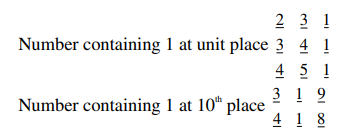# The sum of all 3-digit numbers less than or equal to 500 , that are formed without using the digit "1" and they all are multiple of 11 , is`
Question:

The sum of all 3-digit numbers less than or equal to 500 , that are formed without using the digit "1" and they all are multiple of 11 , is

Solution:

$209,220,231, \ldots, 495$

Sum $=\frac{27}{2}(209+495)=9504$Required $=9501-(231+341+451+319+418)$

7744